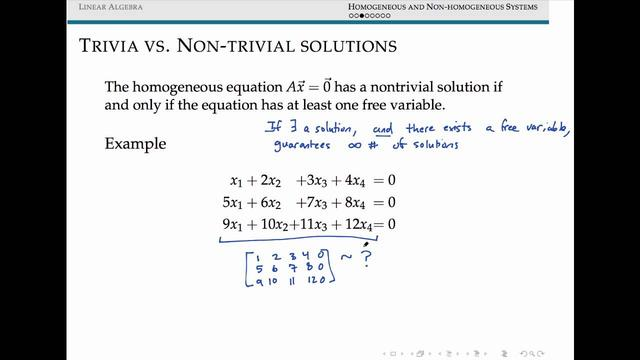# Inhomogeneous linear equations

## Definition

Linear equations whose constant terms are not all zeros are called non-homogeneous linear equations.

The expression of the inhomogeneous linear equations is: Ax=b

## Solution

The steps to solve the inhomogeneous linear equations Ax=b:< /p>(1) Perform elementary row transformation on augmented matrix B into a row ladder shape. If R(A)

(2) If R(A)=R(B), then B is further reduced to the simplest form of the line.

(3) Let R(A)=R(B)=r; use the remaining nr unknowns (free Unknowns) means, and make the free unknowns equal to

section>, you can write a general solution with nr parameters.

## Existence of solutions

Inhomogeneous linear equations

The necessary and sufficient condition for the solution is: the rank of the coefficient matrix is ​​equal to the rank of the augmented matrix, that is, rank(A)=rank(A, b ) (Otherwise, there is no solution).

The necessary and sufficient condition for inhomogeneous linear equations to have a unique solution is rank(A)=n.

The necessary and sufficient condition for inhomogeneous linear equations to have infinitely many solutions is rank(A)

## The structure of the solution

The inhomogeneous linear equations General solution = general solution of homogeneous linear equations + a special solution of non-homogeneous linear equations (η=ζ+η*)

Related Articles